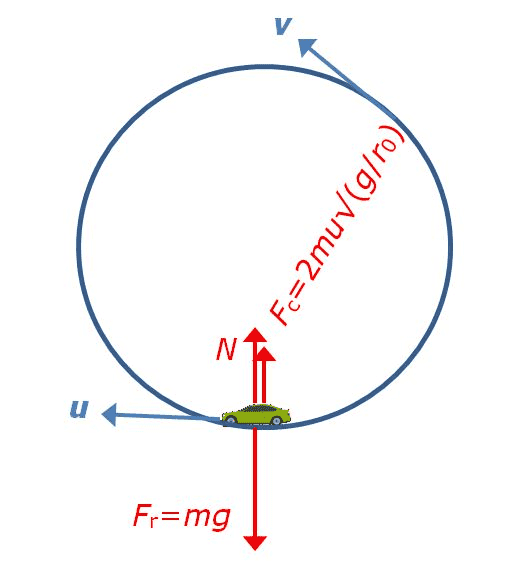# Coriolis force in rotating space station

There is a car driving with speed u opposite the direction of the rotation of the space station. ω=√(g/R) so artificial earth gravity at R. Resulting tangential speed of station is v=√(gR) and centrifugal force is therefore mg radially out. Coriolis force is 2muxω radially in, magnitude 2mu√(g/R). The normal force thus becomes zero when centrifugal force magnitude = coriolis force magnitude or u=½v. My gut tells me it should be u=v. Is this just a case of coriolis force is not always intuitive?Simon Bridge
Homework Helper
You forgot to account for the rotational motion of the car in the rotating reference frame. Bear with me...

The trick is to be careful to do all your maths in one reference frame at a time.

You want to know how fast the car has to go around the station for the normal force at the wheels to be zero.

Take the situation that the car was never spun up with the station in the first place

... in the inertial frame
- there is no normal force, no gravity, no initial speed - that's easy: it stays put.

... in the rotating frame
(Since the normal force is a real force, it will still be the same value (zero) in the rotating frame: which is the condition you want to investigate.)
- the car is acted on by (pseudo)gravity (centrifugal effect) ##F_{pg}## yet it goes in a circle at tangential speed ##v## (which means your intuition is correct): so there must be a net centripetal force ##F_{c}## too. ##F_{c} = F_{cor}-F_{pg}## ... you should be able to work it from there.

The situation you calculated, the car still goes in a circle in the rotating frame, so the normal force cannot be zero - with coriolis and centrifugal forces cancelling, the Normal force is required to provide the centripetal acceleration.

Also see:
https://en.wikipedia.org/wiki/Centrifugal_force#An_equatorial_railway

•f todd baker
Beautiful! Thank you!